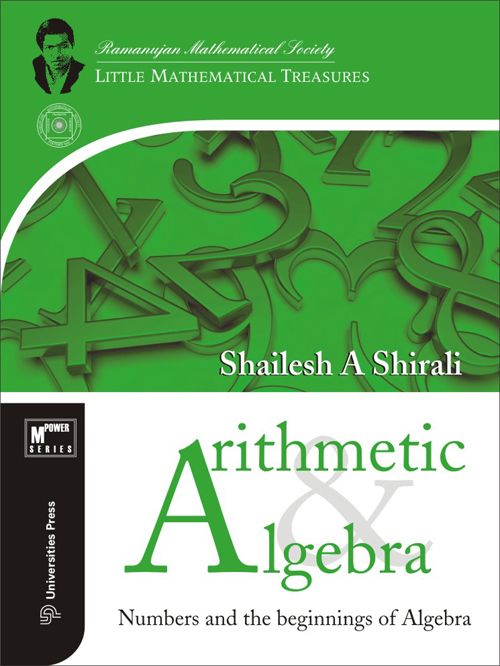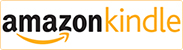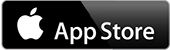## Arithmetic and Algebra: Numbers and the beginnings of Algebra

#### Ebook available on :Arithmetic and Algebra: Numbers and the beginnings of Algebra is written for students of mathematics in classes 7 to 10. It can be used by middle school and high school mathematics teachers who wish to take their students to a deeper level of the subject; it can also be studied by those who have a general interest in the subject. With the help of revealing examples and exercises, the book aims to help students journey into a world of pattern, power and beauty—a journey which can enrich their life greatly.

This is the first book in the MPOWER series of books on arithmetic, algebra and geometry and is included in the Ramanujan Mathematical Series: Little Mathematical Treasures.

#### Contributors (Author(s), Editor(s), Translator(s), Illustrator(s) etc.)

Shailesh Shirali is the Head of the Community Mathematics Centre housed in Rishi Valley School (Andhra Pradesh). He has been deeply involved in the field of mathematics education for three decades, and has been closely associated with the Math Olympiad movement in India.

The series, Mathematical Marvels, authored by him, has been published by Universities Press.

1. The World of Numbers
1.1 The unending supply of numbers
1.2 Roman numerals
1.3 Hindu–Arabic numerals
1.4 Positive and negative
1.6 Multiplication and division
1.7 Number patterns
1.8 Palindromic entertainment
1.9 Miscellaneous problems I
1.10 Miscellaneous problems II
1.11 Miscellaneous problems III

2. Beginnings of Algebra
2.1 Framing sentences
2.2 Expressing patterns
2.3 Laws of algebra
2.4 Using algebra to explain patterns
2.5 Using algebra to discover properties
2.6 Factorisation
2.7 Equations
2.8 Closing quotes

3. Families of Integers
3.1 Odd and even
3.2 Multiples of 3
3.3 Multiples of 5
3.4 Prime and composite
3.5 GCD and LCM
3.6 Euclid’s algorithm
3.7 Perfect numbers
3.8 Squares and cubes
3.9 Triangular numbers
3.10 Powers of 2
3.11 Miscellaneous problems

4. Fractions, Decimals and Percentages
4.1 Fractions as numbers
4.2 Equivalent fractions
4.3 Arithmetic with fractions
4.4 Decimal fractions
4.5 Percentages
4.6 Fractions on the number line
4.7 Rational numbers
4.8 Miscellaneous exercises

5. Fractions: Special Topics
5.1 Recurring decimals
5.2 Powers of integers---on a clothes line!
5.3 Cyclic numbers
5.4 Decimal expansion of 1/p
5.5 Irrational numbers
5.6 Unit fractions
5.7 Mischievous fractions
5.8 Farey sequences
5.9 Miscellaneous exercises

6. Ratio and Proportion
6.1 Ratios for unit conversion
6.2 Rate
6.3 Dimensionless ratios
6.4 Proportion
6.5 Results concerning proportion
6.6 Continued proportion
6.7 Historical note
6.8 Approximations to pi
6.9 The tangram puzzle

7. Indices and Exponents
7.1 Introduction
7.2 The laws of indices
7.3 Big numbers
7.4 The four twos
7.5 Visualising big numbers
7.6 Negative exponents
7.7 Fractional exponents
7.8 Growth patterns
7.9 Exponential notation in science
7.10 Three diversions

8. Polynomials
8.1 Notion of a polynomial
8.2 Degree of the zero polynomial
8.3 Alternate definition
8.4 Multiplication
8.5 Division
8.6 GCD
8.7 Roots of a polynomial
8.8 The remainder theorem
8.9 Symmetric polynomials

9. Expansions in Algebra
9.1 Homogeneous expressions
9.2 Expansions using shortcuts
9.3 Binomial expansions I
9.4 Using the Pascal triangle
9.5 Binomial expansions II
9.6 Squares of multinomials
9.7 Sum and product formulas
9.8 Difference of two squares
9.9 Visual representations
9.10 Miscellaneous expansions
9.11 A claim of Ramanujan’s

10. Factorisation of Polynomials
10.1 Difference of two squares
10.2 Application to integer factorisation
10.3 Factorisation by breaking up an expression
10.5 A more complex example
10.6 Using the factor theorem
10.7 A square investigation

11. Linear Equations
11.1 Introduction to equations
11.2 Genesis of equations
11.3 Solution sets
11.4 Solving an equation
11.5 Linear equations in two unknowns
11.6 Application I: Clock problems
11.7 Application II: Pythagorean triples
11.8 Application III: Squares in AP
11.9 Application IV: Unit fractions

12. Problem Corner
12.1 Appetisers
12.2 For the junior mathlete

13. Investigations
13.1 Mischievous cancellations
13.2 Mischievous square roots
13.3 Prime numbers
13.4 Digital sums
13.5 Digital reversals
13.6 Squares and 2006 . . .
13.7 Squares and cubes
13.8 Still more squares
13.9 And yet more squares . . .
13.10 Accommodating numbers